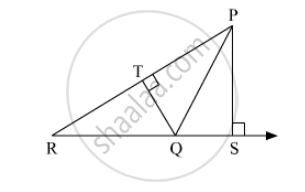Advertisement Remove all ads

# In Adjoining Figure, Seg Ps ⊥ Seg Rq Seg Qt ⊥ Seg Pr. If Rq = 6, Ps = 6 and Pr = 12, Then Find Qt. - Geometry

Sum

In adjoining figure, seg PS ⊥ seg RQ seg QT ⊥ seg PR. If RQ = 6, PS = 6 and PR = 12, then Find QT.Advertisement Remove all ads

#### Solution

Given:
PS ⊥ RQ
QT ⊥ PR
RQ = 6, PS = 6 and PR = 12

With base PR and height QT, A(Δ PQR) = 1/2 xx "PR" xx "QT"

With base QR and height PS, A(Δ PQR) =1/2 xx  "QR" xx "PS"

Let we know that "The ratio of areas of two triangles is equal to the ratio of the product of their base and corresponding heights."

⇒ In Δ PQR base RQ , heights = PS

("A" (triangle "PQR"))/("A" (triangle "PQR")) = (1/2xx"PR"xx"QT")/(1/2xx"QR"xx"PS")

=> 1 = ("PR"xx"QT")/("QR"xx"PS")

=> "PR"xx"QT" = "QR"xx"PS"

QT = ("QR"xx"PS")/"PR"

= (6 xx 6)/12

= 36/12

QT = 3/1

Hence, the measure of side QT is 3 units.

Concept: Similar Triangles
Is there an error in this question or solution?
Advertisement Remove all ads

#### APPEARS IN

Balbharati Mathematics 2 Geometry 10th Standard SSC Maharashtra State Board
Chapter 1 Similarity
Practice Set 1.1 | Q 3 | Page 6
Advertisement Remove all ads
Advertisement Remove all ads
Share
Notifications

View all notifications

Forgot password?